# Constellation Compaction Design to Reduce Both PAPR and Average Power in OFDM Systems

## Abstract

This paper proposes a novel way to compact the constellation points in QAM modulation schemes and exploits this compaction to reduce the peak to average power ratio (PAPR) in orthogonal frequency division multiplexing (OFDM) systems. The compaction is accomplished using one dimensional product code (PC). Significant PAPR reduction is achieved by carefully mapping the points in the compact version of the constellation to their radial symmetric points and choosing for transmission signalling points which result in lower PAPRs. Specifically, the complementary cumulative distribution function (CCDF) of the modified OFDM signal shows about five dB improvement in PAPR at a clipping probability of 10−4 over the original OFDM signal. This performance is comparable to that in similar PAPR reduction schemes; however, it is achieved using simple single parity check (SPC) product code and at lower average power.

## 1. Introduction

At high data rates and large bandwidth, communication channels become frequency selective. Single carrier modulation schemes do not perform well under this condition, which has necessitated a shift to multicarrier modulation schemes. Multicarrier schemes such as orthogonal frequency division multiplexing (OFDM) transform a frequency selective fading channel into a flat fading channel, simplifying or even eliminating equalization. This parallel data transfer method offers immunity to multipath fading and impulsive noise and can be efficiently implemented in digital hardware through the use of fast Fourier transform (FFT) [1, 2].

Despite these advantages, one major drawback is that OFDM signal exhibits high peak-to-average power ratio (PAPR). Such large dynamic range of these signals, when amplified, drive the high-power amplifier to operate in the nonlinear region. This results in two types of distortions: out of-band distortion which manifests itself as an increase in adjacent channel interference (ACI) and in-band distortion which manifests itself as an increase in bit error rate (BER) . These effects of nonlinearities on OFDM transmissions have been investigated in different publications using different metrics such as spectrum regrowth and BER degradation [3, 4]. The CCDF of the PAPR for the transmitted OFDM signal is the most commonly used performance metric. The CCDF provides a graphical indication of the probability of the OFDM signal envelope, within the OFDM frame, exceeding a specified PAPR threshold,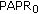, and it is this performance metric that is adopted in this paper.

There are many PAPR reduction methods in the literature . These methods trade off complexity, data rate, signal power, and BER to reduce the high PAPR of the transmitted OFDM signal. We will discuss some of these methods that are closely related to the work in this paper. Tone injection (TI) method extends, in each subcarrier, the conventional QAM constellation by translating the original constellation and partitions these extensions into cosets [8, 9]. When large peaks are present, the extra cosets can be used to pick alternative constellation points to reduce the PAPR. Radial symmetric (RS) method maps a point in the original constellation to its symmetric point in an outer circle . The radius of this outer circle is chosen to make sure that the minimum distance,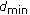, in the original constellation is not compromised in the new expanded constellation. The above methods reduce the PAPR at the expense of increasing the average power. Coding techniques reduce the high PAPR by mapping the data symbols to codewords or phases that give the minimum PAPR from a given set of codewords or phases. However, finding the codewords with low PAPR requires an exhaustive search for optimal codewords or phases [5, 11]. Error insertion (EI) method is a coding technique in which subcarriers are changed by intentionally inserting errors in these subcarriers in such a way that the resultant modified OFDM signal will have a reduced PAPR . These errors are corrected in the receiver by decoding. Although this method does not compromise the average power of the system, it uses a complex coding technique like BCH codes and needs to change a relatively large number of subcarriers to accomplish a good PAPR reduction.

In this paper, we introduce an approach to compact the QAM constellation which resulted in a reduction in the average power. The compaction is achieved by using a simple single parity check product code (SPCPC) which limits the constellation points to certain ones. After constellation compaction, the PAPR is reduced by mapping each point in the compact form to its radial symmetric point, which is shown in  to be the best mapping location, using two methods. Method I maps the points in the compact version of the constellation to the unused points in the original constellation. Method II maps the compact points to their radial symmetric points on an external circle. These proposed methods provide very good reduction by compromising coding gain, average power increase, and complexity.

## 2. PAPR and OFDM Systems Fundamentals

Consider the baseband-equivalent OFDM transmitter with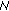subcarriers in whichmodulation symbols,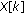, are mapped to the continuous time OFDM signal,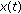, as follows: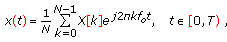(1)

where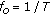and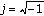. Modulation symbolsusually come from PSK or QAM constellation points. The discrete time version of (1), referred to as the OFDM frame, is formed by samplingat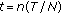to get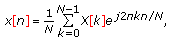(2)

where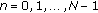. In (2), the OFDM symbols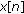are related to modulation symbolsthrough an inverse discrete Fourier transform (IDFT) efficiently calculated using the inverse fast Fourier transform (IFFT).

The continuous PAPR,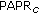, ofis the ratio of the maximum instantaneous power to the average power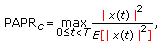(3)

where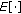is the expectation operator. The discrete PAPR ofnoted by just PAPR is given by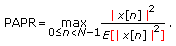(4)

The PAPR of an oversampled version ofcalculated as in (4) provides an accurate approximation of theas in (3) provided that the oversampling factor is at least four . Also, a cyclic prefix is typically pre-pended to the OFDM symbol in the transmitter and removed in the receiver. Cyclic prefix is introduced in order to alleviate the effects of ISI and interchannel interference (ICI). This cyclic prefix does not affect the PAPR of the OFDM symbol and consequently is not considered in this paper .

In a simplified version of an OFDM system, the serial information bit stream is converted into groups of parallel streams using serial-to-parallel (S/P) converter. The parallel groups of bits are encoded and/or modulated and then pass through the IFFT. The outputs of the IFFT are converted into a serial stream using the parallel-to-serial (P/S) converter to get the discrete time version of the OFDM signal. A cyclic prefix is introduced with the same length as the channel impulse response to make the OFDM signal. The discrete time OFDM signal is converted to an analog signal using digital-to-analog converter, amplified using HPA, and sent through the channel in which noise is encountered. At the receiver, the reverse process is done to get back the original transmitted information.

We present a common framework shown in Figure 1 used in many methods to reduce the PAPR in OFDM systems. These methods are generally based on introducing perturbations into the original modulation symbols so that the time domain OFDM frame will have a reduced PAPR. In PAPR reduction methods based on multiple-point symbol representations, valid perturbations to the original modulation symbols on different subcarriers are generated first and then the corresponding PAPRs are calculated. The OFDM frame with the minimum PAPR is actually chosen for transmission into the channel. This process is visualized in Figure 1 where the perturbation generator synthesizes alternative representations of the original symbols and the peak acceptor checks for the minimum PAPR.

Using time-frequency equivalence, computationally efficient algorithms have been developed for this kind of PAPR reduction methods . Rather than using the exhaustive search to simultaneously change more than one subcarriers, a sequential search is recommended. On the first pass of the sequential search, only one signalling point amongsubcarriers is optimized and then fixed along with its corresponding subcarrier. On the next pass of the sequential search, another signalling point is attempted with the first one fixed and so on . This is the implementation pursued in this paper.

## 3. Constellation Compaction

In conventional OFDM systems, the S/P converter converts the serial bits intogroups of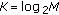bits, whereand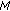are the number of subcarriers and the number of constellation points in the modulation scheme, respectively. In our approach, the S/P converts the serial bits intogroups of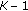bits. The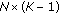bits are then encoded using one-dimensional product code (1D PC). Basically, as shown in Figure 2, the data (bits) are put in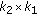matrix form where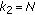and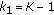in our case. Then the encoding using SPC code is done row-wise only using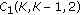to form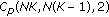product code. This makes the rate of the SPC code and the overall 1D SPCPC to be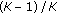.

As mentioned in the introduction, coding [14, 15] is used to reduce PAPR by compromising its gain, that is, part of the coding gain is devoted to reduce PAPR. Here, we get advantage of the parallel structure of the OFDM system and use product code that forces the signalling points of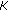bits to take values at certain locations in the constellation. In Figure 3, 16-ary QAM modulation using Gray coding is shown. The inner eight points have the second least significant bit (SLSB) equals one. By forcing the SLSB of each group of 4-bit coming from the encoder to one, we modulate the bits (symbols) to one of the eight points inside the dashed rectangle. We call this process constellation compaction. This is equivalent to forcing the second column of the PC to one which is actually introducing one error in half of the rows, on average, of the PC. Since the location of the error, if any, at each row is known, it is actually an introduction of one erasure in that row. This erasure introduction in the OFDM transmitter can be recovered at the receiver by erasure decoding. In erasure decoding, the second column of the PC is erased at the decoder. Then, it is recovered by simply modulo-two addition of the remaining columns. If, at the encoder, the redundancy bit for each group is positioned at the second column of the product code (the SLSB position), the recovery process, at the decoder, can be further simplified. In this case, erasure decoding is performed by just erasing the second column, and there is no need to recover it back since it corresponds to redundant bits. After constellation compaction, it is required to reduce the high PAPR of the OFDM signal. The search model in Figure 1 described in Section 2 is followed. Now, how a subcarrier is changed to give a smaller PAPR? This is explained in the next section.

## 4. PAPR Reduction Methods

The high PAPR of the OFDM signal can be reduced by taking advantage of the compact constellation and the concept of radially symmetric mapping. It is shown in  that the maximum PAPR reduction is achieved when a point in the constellation is mapped to its radial symmetric point. Based on this premise, we introduce two methods to reduce the high PAPR. Method I maps the inner points in the 16-ary QAM constellation to the outer unused points. We show two variations of this method depending on the type of coding used for the bits: Gray coding and non-Gray coding. Gray coding is shown in Figure 3 for 16-ary QAM modulation. For the readability of the figure, we show the mapping of only the upper inner points to the lower outer points. The other half of the points are mapped in the same manner. After demodulation, we must make sure that all the demodulated points correspond to only the inner eight points. If a demodulated point corresponds to one of the outer eight points, then it is actually a subcarrier that the perturbation generator in Figure 1 changes (maps it to its radial symmetric point) to reduce the PAPR. Therefore, we need to map all such points after performing demodulation to their corresponding inner points before we can decode since these points differ from their radial inner points in not only the SLSB.

In the second variation of Method I, we map the inner points into the outer points but using non-Gray coding as shown in Figure 4. For the readability of the figure, we show again only the mapping of half of the points. This 16-ary QAM modulation using non-Gray coding is not optimum since it is known that the optimum BER is obtained using Gray coding. However, the increase in the BER is very minimal since we are just changing few subcarriers (points). The advantage of this variation is that each outer point differs from its corresponding inner point in the SLSB which simplifies erasure decoder even more. At the receiver, we just apply demodulation followed by decoding where decoding is performed by just erasing the SLSB bit. We use the first variation of Method I. We introduce the second variation just to show that we can compromise BER and demodulation/decoding complexity.

Method II takes advantage of the exact radial symmetric mapping which is shown in Figure 5. For the readability of the figure, we show only the mapping of half of the points. In this figure, each point in the internal points is mapped into its radial symmetric point on the circle having a radius greater than the highest point in the original constellation by at leastin the original constellation. Also, we have to make sure that the distance between any two points on the circle to be at least. This is to ensure that the BER of the modulation scheme is not compromised. For this method, we use the radius which is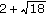where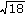is the distance from the origin to the farthest point in the constellation and 2 is the minimum distance in the original 16-ary QAM modulation. By this choice, we can compare Method II with the RS method in  which assumes the same radius. At the receiver, any point that is detected to be on the circle is mapped back into its radial symmetric inner point and then the SLSB is erased followed by erasure decoding to recover the missing bits (columns).

For higher modulation scheme such as 64-ary QAM modulation, Method I can be applied directly while Method II can be applied with some modification. Since, in higher modulation schemes, a point on the circle may correspond to more than one point in the internal points, we can fix (no mapping) some point in the internal points and we change only some points that have higher value in the internal points. This was shown to be valid in . The very internal points in the constellation were shown to contribute almost negligibly in the PAPR reduction .

## 5. Results and Discussion

The performance of the OFDM system that employs the QAM constellation compaction discussed in Section 3 and uses the methods discussed in Section 4 is evaluated using CCDF. We simulated 100,000 OFDM frames. We consider OFDM signal of 64 subcarriers (tones) and an oversampling factor of four. All the values presented in this section are taken at a clipping probability of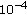. Figure 6 shows the performance of Method I and the comparison to the closely related method which is the EI method. Method I achieves five dB gain after changing five tones which is the same gain the EI method provides after changing six tones with complex BCH encoding/decoding. Figure 7 shows the performance of Method II and the comparison to the closely related methods which are the TI and the RS methods. Method II outperforms both RS and TI methods by approximately 0.4 dB after changing three tones. After changing only four tones, Method II achieves greater than five dB gain and therefore outperforms the EI method that achieves approximately the same gain after changing six tones. We find that Method I needs one extra tone to change to achieve the same performance as Method II. However, the overall average power reduction for Method I, as will be seen shortly, is greater than Method II. Therefore, the choice between these two method becomes an application dependent by trading average power and complexity.

Table 1 shows the overall average power increase for the proposed methods using 16-ary QAM OFDM signals. The negative sign for Method I and Method II indicates a reduction in the average power. We observe that EI method does not increase or reduce the average power of the system. RS and TI methods increase the average power of the system and the increase using TI is larger than that of RS. However, our two proposed methods with the concept of constellation compaction actually reduce the overall average power of the OFDM system. After five tones changes, we observe approximately 1.8 dB and 0.8 dB reductions using Method I and Method II, respectively. As the number of tone changes increases, the difference in average power reduction between Method I and Method II increases in favor of Method I. It should be emphasized here that the two methods provides very good reduction of PAPR using very simple encoding/ decoding process involving SPCPC at the expense of a slight reduction of data rate because of the use of coding. The rate of the code needed is very high especially for higher constellation modulation and is given by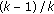. Both methods do not compromise the BER of the modulation scheme used since it keeps the same.

It should be emphasized, as well, that the figures for the average power increase/reduction are the maximum/minimum average power increase/reduction when we attempt to change the tone(s) for each and every OFDM frame. However, it is noted that the percentage of the tone(s) usage is apparently less than 100 % which means that for some frames the tone(s) are not changed because such frames already have the minimum PAPR. Therefore, to find the actual average power increase/reduction, we have to multiply the figures in Table 1 with the percentage of tone usage. Moreover, in a real system, in order to reduce the average power, one may choose not to attempt to change the tone(s) for every block but when the PAPR reduction due to the signalling point change reaches a predetermined threshold. Also, when we change some tones and find that the reduction is actually very small, it can be discarded. This means that the actual percentage usage of the tones will be even smaller and hence the actual increase/reduction in the average power is smaller/bigger as well.

## 6. Conclusion

This paper provides two methods to reduce the high PAPR in OFDM systems utilizing the radial symmetry concept. The reduction is based on exploiting part of the coding gain of a simple single parity check product code. This code results in a compact constellation since the constellation points after encoding is limited to half of the constellation points. The compaction reduced the average power by about 2.22 dB when compared to the noncompact 16-ary QAM constellation. The reduction is achieved by introducing one erasure in each row of the product code. These erasures are recovered by simple modulo-two addition along the rows of the product code. Method I maps the compact points to the unused points in the original noncompact constellation. Method II maps the compact points to their exact radial symmetric points on the circle with a radius maintaining theof original constellation. Using Method I, five dB PAPR reduction is achieved after changing five tones whereas the same performance is obtained using Method II after changing only four tones. However, Method I achieved this performance at lower average power compared to Method II. The performance of these two methods are slightly better than similar techniques such as error insertion and radial symmetric methods but it is achieved using low complexity single parity check product code. The usage of coding reduces the date rate of the systems, however, this reduction is very small since the code rate is very high especially for high-modulation schemes.

## References

1. Han SH, Lee JH: An overview of peak-to-average power ratio reduction techniques for multicarrier transmission. IEEE Wireless Communications 2005, 12(2):56-65. 10.1109/MWC.2005.1421929

2. Li Y, Stuber GL: Orthogonal Frequency Division Multiplexing for Wireless Communications. Springer, New York, NY, USA; 2006.

3. Tellado J, Cioffi J: PAR reduction in multicarrier transmission systems. ITU; February 1998.

4. Verma A, Arvind MT: Peak to average power ratio reduction in multicarrier communication systems. Proceedings of the IEEE International Conference on Personal Wireless Communications, February 1999 1: 204-206.

5. Davis JA, Jedwab J: Peak-to-mean power control in OFDM, Golay complementary sequences, and Reed-Muller codes. IEEE Transactions on Information Theory 1999, 45(7):2397-2417. 10.1109/18.796380

6. Li X, Cimini LJ: Effects of clipping and filtering on the performance of OFDM. IEEE Communications Letters 1998, 2(5):131-133. 10.1109/4234.673657

7. Müller SH, Huber JB: OFDM with reduced peak-to-average power ratio by optimum combination of partial transmit sequences. Electronics Letters 1997, 33(5):368-369. 10.1049/el:19970266

8. Hwang CS: A peak power reduction method for multicarrier transmission. Proceedings of the International Conference on Communications (ICC '01), June 2000 5: 1496-1500.

9. Narula A, Kschischang F: Unified framework for PAR control in multitone systems. ANSI Document June 1998., (T1E1.4 no. 98–183):

10. Al-Shaikhi AA, How J: Alternative symbol representations with radial symmetry for PAPR reduction in OFDM systems. Proceedings of the IEEE International Conference on Communications (ICC '07), June 2007, Glasgow, UK 2942-2948.

11. Wilkinson TA, Jones AE: Minimisation of the peak to mean envelope power ratio of multicarrier transmission schemes by block coding. Proceedings of the 45th IEEE Vehicular Technology Conference (VTC '95), July 1995 825-829.

12. Jamieson C, Ilow J: Bit mapping and error insertion for FEC based PAPR reduction in OFDM signals. Proceedings of the IEEE Global Telecommunications Conference (GLOBECOM '06), December 2006, San Francisco, Calif, USA 1-5.

13. Tellambura C: Computation of the continuous-time PAR of an OFDM signal with BPSK subcarriers. IEEE Communications Letters 2001, 5(5):185-187. 10.1109/4234.922754

14. Jones AE, Wilkinson TA, Barton SK: Block coding scheme for reduction of peak to mean envelope power ratio of multicarrier transmission schemes. Electronics Letters 1994, 30(25):2098-2099. 10.1049/el:19941423

15. Fischer RFH, Siegl C: Reed-Solomon and simplex codes for peak-to-average power ratio reduction in OFDM. IEEE Transactions on Information Theory 2009, 55(4):1519-1528.

16. Al-Shaikhi AA, Ilow J: Erasure based PAPR reduction using minimally expanded constellations in OFDM systems. Proceedings of the 50th Annual IEEE Global Telecommunications Conference (GLOBECOM '07), November 2007, Washington, DC, USA 2904-2909.

## Acknowledgment

A. Al-Shaikhi acknowledges the support of King Fahd University of Petroleum and Minerals (KFUPM).

## Author information

Authors

### Corresponding author

Correspondence to Ali Al-Shaikhi.

## Rights and permissions

Reprints and Permissions

Al-Shaikhi, A. Constellation Compaction Design to Reduce Both PAPR and Average Power in OFDM Systems. J Wireless Com Network 2010, 304951 (2010). https://doi.org/10.1155/2010/304951

• Revised:

• Accepted:

• Published:

• DOI: https://doi.org/10.1155/2010/304951

### Keywords

• Orthogonal Frequency Division Multiplex
• Orthogonal Frequency Division Multiplex System
• Cyclic Prefix
• Orthogonal Frequency Division Multiplex Symbol
• Orthogonal Frequency Division Multiplex Signal